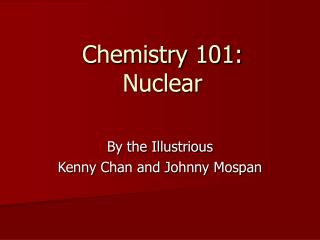# Chemistry 101: Nuclear - PowerPoint PPT PresentationDownload PresentationChemistry 101: Nuclear

Chemistry 101: NuclearDownload Presentation## Chemistry 101: Nuclear

- - - - - - - - - - - - - - - - - - - - - - - - - - - E N D - - - - - - - - - - - - - - - - - - - - - - - - - - -
##### Presentation Transcript

1. Chemistry 101:Nuclear By the Illustrious Kenny Chan and Johnny Mospan

2. Decay Particles • Alpha Particles (α) – He-2 • Beta Particles (β) - e- • Photon Particles (γ)

3. Alpha Decays • U-238 → He-4 + ___? • The # of protons and mass must be equal on both sides. • Therefore, the unknown must have 90 protons • And since Uranium has a mass of 238 and Helium has a mass of 4, the unknown has a mass of 234.

4. Alpha Decays (cont.) • U-238 → He-4 + ___? • Well, the unknown is Th-90 • U-92 → He-2 + Th-90

5. Beta Decays • Th-91 → β + ____? • Same logic as before. Thus, 91 protons for the unknown. • Furthermore, since Thallium has a mass of 234 and a Beta particle has a mass of 0, then the unknown has a mass of 234. • The unknown is Pa-234 • Th-91 → β + Pa-234

6. Positron Emission • A positron is a nuclear particle having the same mass as an electron but a positive charge. • Same concept as Beta decays, except the Beta particle has a charge of 1+ instead of 1-

7. Positron Emission (cont.) • Po-207 → β (1+) + ____? • Same logic as before. • Since Polonium has 84 protons, the unknown must have 83 protons. • Mass of unknown must be 207 • The unknown is Bi-207 • Po-207 → β (1+) + Bi-207

8. e- Capture • It’s basically the opposite of a Beta decay • Put the Beta particle on the reactants side instead of on the products side • Be-7 + β → ____? • Same logic as before. • Unknown must have 3 protons. • Unknown must have a mass of 7 • Be-7 + β → Li-7

9. More Decay Stuff • Johnny’s an idiot and can’t scan stuff. • Johnny’s also lazy. • See board for picture.

10. Mass Defect • Given: • H-1 + n-1 → H-2 • H-1 = 1.004552g • n-1 = 1.008665g • H-2 = 2.01410g • Find Change in Energy.

11. Mass Defect (cont.) • ∑ mass (final) - ∑ mass (initial) = Δm • (2.01410) – (1.007825 + 1.008665) = Δm • Δm = -0.00239 g/mol • E = (Δm) c^2 • C (speed of light… aka “Johnny’s constant) = 2.9979 * 10^8 • E = (0.00239)(3*10^8) = 2.15*10^8 J/mol

12. Mass Defect (cont.) • In fusion, mass defect is associated with binding energy • Fusion is putting things together to form one product • In fission, mass defect is associated with kinetic energy of product particle • Fission is splitting things apart from one reactant

13. Rate of Nuclear Decays • A = Activity = decays/time • A = kN • Derive equation for Number of Decays at time T (see board!)

14. Half-Life Stuff • At half-life, 2N = No • Derive equation for half-life… • (see board!)

15. Predicting Decays • Once again, see the board.

16. The End. • Mospan and Chan 1, Chemistry 0